# Possible lengths

Find the most possible lengths for the third side of a triangle with sides 20 and 18.

Result

c = (Correct answer is: 2 < c < 38)#### Solution:

$a=20 \ \\ b=18 \ \\ \ \\ a+b > c \ \\ a+c > b \ \\ b+c > a \ \\ \ \\ 20+18 >c \ \\ 20+c >18 \ \\ 18+c >20 \ \\ \ \\ 38 > c \ \\ -2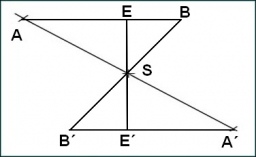We would be pleased if you find an error in the word problem, spelling mistakes, or inaccuracies and send it to us. Thank you!Tips to related online calculators
Do you want to convert length units?

#### You need to know the following knowledge to solve this word math problem:

We encourage you to watch this tutorial video on this math problem:

## Next similar math problems:

• Triangles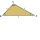Five sticks with a length of 2,3,4,5,6 cm. How many ways can you choose three sticks to form three sides of a triangle?
• Triangles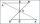Hanka cut the 20 cm long straws into three pieces each piece had a length in cm. Then, with these three pieces, she tried to make a triangle. a) What circuit has each of the triangles? b) How long can the longest side measure? c) How many different triang
• TrianglesIvo wants to draw all the triangles whose two sides of which have a length of 4 cm and 9 cm, and the length of the third side is expressed in whole centimeters. How many triangles does he have?
• TriangleProve whether you can construct a triangle ABC, if a=9 cm, b=6 cm, c=10 cm.
• 3-bracket 2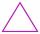May be the smallest angle in the triangle greater than 70°?
• The perimeter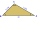The triangle has one side 5 cm long and the another 11 cm long. What can be the smallest and what is the largest perimeter?